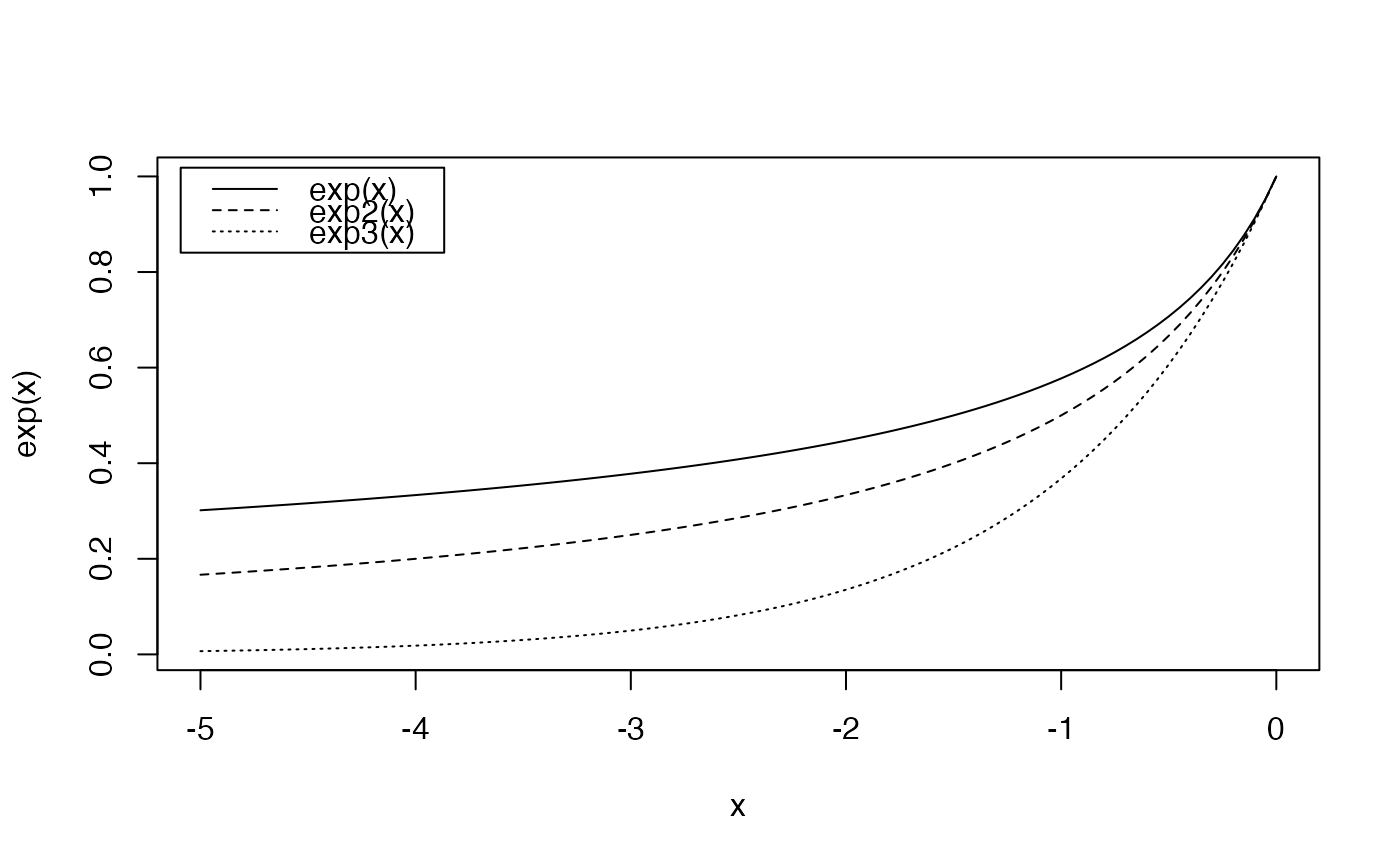Calculates the deformed exponential of order $$q$$.

expq(x, q)
expq.CommunityProfile(Profile)

## Arguments

x

A numeric vector.

Profile

A CommunityProfile.

q

A number.

## Details

The deformed exponential is defined as $$(x(1-q)+1)^{\frac{1}{(1-q)}}$$.

For $$q>1$$, $$\ln_q{(+\infty)}=\frac{1}{(q-1)}$$ so $$\exp_q{(x)}$$ is not defined for $$x>\frac{1}{(q-1)}$$.

expq.CommunityProfile calculates the deformed exponential of a CommunityProfile. Its $x item (the order of dversity) is kept unchanged whilst other items are set to their exponential of order $x. Thus, an entropy profile is transformed into a diversity profile.

## Value

A vector of the same length as x containing the transformed values or a CommunityProfile.

expq
curve(exp(x), -5, 0, lty=3)